#Interactive Real Analysis

## 6. Limits, Continuity, and Differentiation

### 6.3. Discontinuous Functions

 Definition 6.3.1: Discontinuous function If a function fails to be continuous at a point c, then the function is called discontinuous at c, and c is called a point of discontinuity, or simply a discontinuity.
Points of discontinuity can be classified into three different categories: 'fake' discontinuities, 'regular' discontinuities, and 'difficult' discontinuities.
 Examples 6.3.2: Consider the following three functions: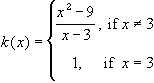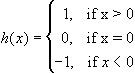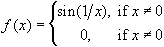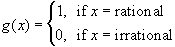Which of these functions, without proof, has a 'fake' discontinuity, a 'regular' discontinuity, or a 'difficult' discontinuity ?
Of course, we need some mathematical description of the various types of discontinuities that a function could have.
 Definition 6.3.3: Classification of Discontinuities Suppose f is a function with domain D and cD is a point of discontinuity of f. if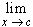f(x) exists, then c is called removable discontinuity. iff(x) does not exist, but both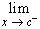f(x) and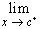f(x) exit, then c is called a discontinuity of the first kind , or jump discontinuity if eitherf(x) orf(x) does not exist, then c is called a discontinuity of the second kind, or essential discontinuity.
 Examples 6.3.4: Consider the functions from the previous examples. ThenProve that k(x) has a removable discontinuity at x = 3, and draw the graph of k(x).Prove that h(x) has a jump discontinuity at x = 0, and draw the graph of h(x)Prove that f(x) has a discontinuity of second kind at x = 0What kind of discontinuity does the function g(x) have at every point (with proof).
It is clear that any function is either continuous at any given point in its domain, or it has a discontinuity of one of the above three kinds. It is also clear that removable discontinuities are 'fake' ones, since one only has to define f(c) =f(x) and the function will be continuous at c. Of the other two types of discontinuities, the one of second kind is hard. Fortunately, however, discontinuities of second kind are rare, as the following results will indicate.
 Definition 6.3.5: Monotone Function A function f is monotone increasing on (a, b) if f(x)f(y) whenever x < y. A function f is monotone decreasing on (a, b) if f(x)f(y) whenever x < y. A function f is called monotone on (a, b) if it is either always monotone increasing or monotone decreasing.
Note that f is increasing if -f is decreasing, and visa versa. Equivalently, f is increasing if
• f(x) / f(y)1 whenever x < y
• f(x) - f(y)0 whenever x < y
These inequalities are often easier to use in applications, since their left sides take a very nice and simple form. Next, we will determine what type of discontinuities monotone functions can possibly have. The proof of the next theorem, despite its surprising result, is not too bad.
 Theorem 6.3.6: Discontinuities of Monotone Functions If f is a monotone function on an open interval (a, b), then any discontinuity that f may have in this interval is of the first kind. If f is a monotone function on an interval [a, b], then f has at most countably many discontinuities.
This theorem also states that if a function wants to have a discontinuity of the second kind at a point x = c, then it can not be monotone in any neighborhood of c.
 Corollary 6.3.7: Discontinuities of Second Kind If f has a discontinuity of the second kind at x = c, then f must change from increasing to decreasing in every neighborhood of c.
In other words, f must look pretty bad if it has a discontinuity of the second kind.
 Examples 6.3.8:What kind of discontinuity does the function f(x) = exp(1/x) have at x = 0 ?What kind of discontinuity does the function f(x) = x sin(1/x) have at x = 0 ?What kind of discontinuity does the function f(x) = cos(1/x) have at x = 0 ?